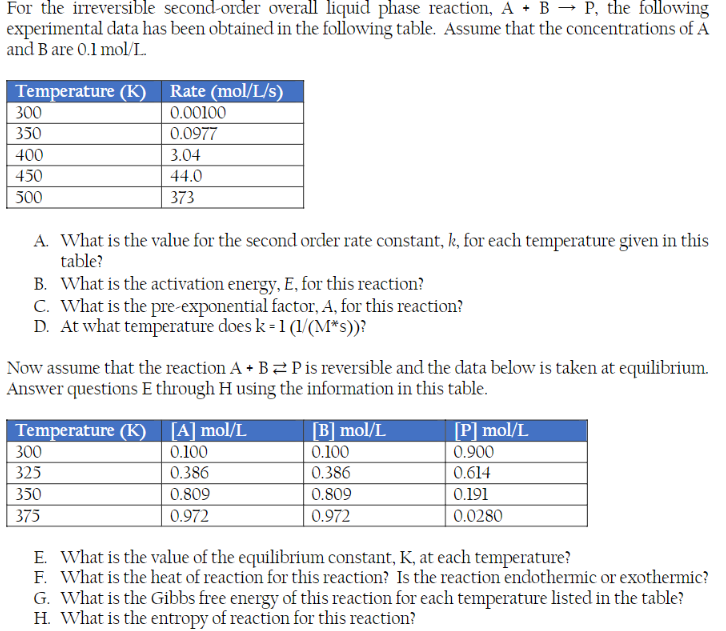Home / Expert Answers / Chemical Engineering / for-the-irreversible-second-order-overall-liquid-phase-reaction-mathrm-a-mathrm-b-rightar-pa282

# (Solved): For the irreversible second-order overall liquid phase reaction, $$\mathrm{A}+\mathrm{B} \rightar ...For the irreversible second-order overall liquid phase reaction, \( \mathrm{A}+\mathrm{B} \rightarrow \mathrm{P}$$, the following experimental data has been obtained in the following table. Assume that the concentrations of $$\mathrm{A}$$ and $$\mathrm{B}$$ are $$0.1 \mathrm{~mol} / \mathrm{L}$$ A. What is the value for the second order rate constant, $$k$$, for each temperature given in this table? B. What is the activation energy, $$E$$, for this reaction? C. What is the pre-exponential factor, $$A$$, for this reaction? D. At what temperature does $$k=1\left(1 /\left(M^{*} s\right)\right)$$ ? Now assume that the reaction $$\mathrm{A}+\mathrm{B} \rightleftarrows \mathrm{P}$$ is reversible and the data below is taken at equilibrium. Answer questions E through $$\mathrm{H}$$ using the information in this table. E. What is the value of the equilibrium constant, $$\mathrm{K}$$, at each temperature? F. What is the heat of reaction for this reaction? Is the reaction endothermic or exothermic? G. What is the Gibbs free energy of this reaction for each temperature listed in the table? H. What is the entropy of reaction for this reaction?

We have an Answer from Expert